# Cauchy distribution

2010 Mathematics Subject Classification: Primary: 60E07 [MSN][ZBL]

A continuous probability distribution with densityand distribution function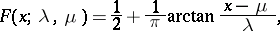whereand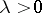are parameters. The Cauchy distribution is unimodal and symmetric about the point, which is its mode and median. No moments of positive order — including the expectation — exist. The characteristic function has the form. The class of Cauchy distributions is closed under linear transformations: If a random variablehas the Cauchy distribution with parametersand, then the random variable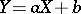also has a Cauchy distribution, with parameters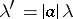and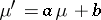. The class of Cauchy distributions is closed under convolution:(*)in other words, a sum of independent random variables with Cauchy distributions is again a random variable with a Cauchy distribution. Thus, the Cauchy distribution, like the normal distribution, belongs to the class of stable distributions; to be precise: It is a symmetric stable distribution with index 1 (cf. Stable distribution). The following property of Cauchy distributions is a corollary of (*): If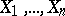are independent random variables with the same Cauchy distribution, then their arithmetic mean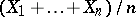has the same distribution as each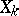. One more property of Cauchy distributions: In the family of Cauchy distributions, the distribution of a sum of random variables may be given by (*) even if the variables are dependent. For example, ifand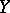are independent and have the same Cauchy distribution, then the random variablesand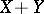have the same Cauchy distribution. The Cauchy distribution with parametersand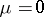is the Student-distribution with one degree of freedom. The Cauchy distribution with parameters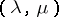is identical with the distribution of the random variable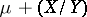, whereandare independent and normally distributed with parameters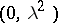and, respectively. A random variable with this distribution is the function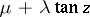, whereis a random variable uniformly distributed on the interval. The Cauchy distribution is also defined in spaces of dimension greater than one. The concept was first investigated by A.L. Cauchy.